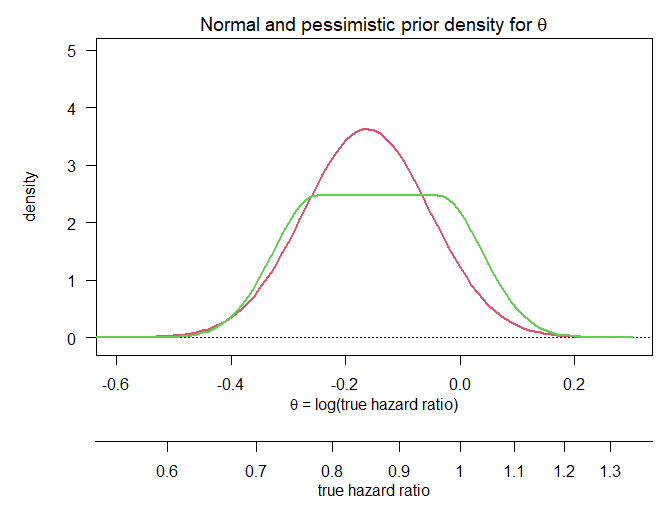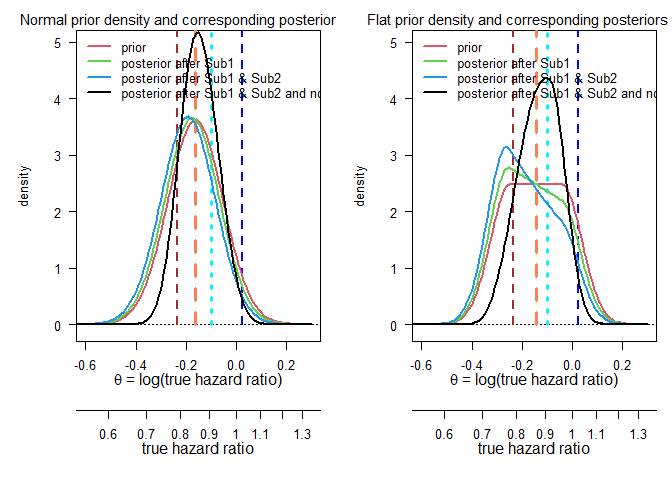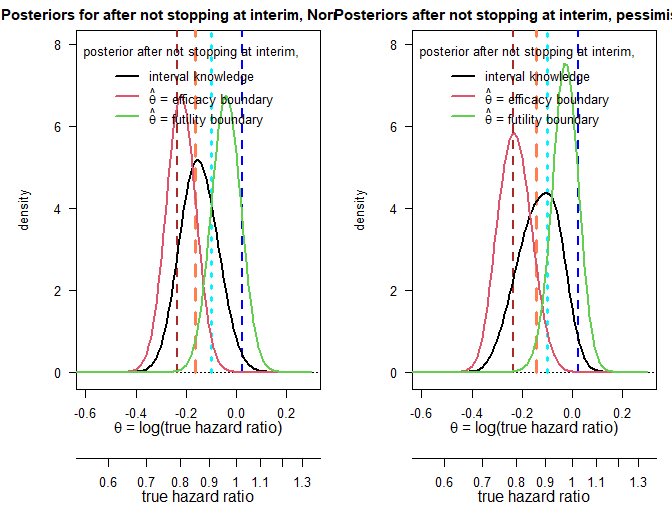# Sequentially updating the likelihood of success of a Phase 3 pivotal time-to-event trial based on interim analyses or external information

Department of Biostatistics, Roche Basel

# Purpose

This document reproduces the computations in Rufibach, Jordan, and Abt (2016b) (doi). All the references below to sections, figures, or tables are with respect to that paper. The functions used are all implemented in the R package Rufibach, Jordan, and Abt (2016a) and all the code below is also available in the help file of the function bpp_1interim.

Further discussions around Bayesian Predictive Power (BPP), mainly about the choice of a prior, are provided in Rufibach, Burger, and Abt (2016) (doi).

# Setup

We start with defining the parameters of the pivotal trial and the results from the two external studies:

# load the package:
library(bpp)

# get the code for all the computations below:
?bpp_1interim

# specifications of the the pivotal trial
propA <- 0.5   # proportion of patients randomized to arm A
fac <- (propA * (1 - propA)) ^ (-1)
nevents <- c(0.5, 1) * 1600
finalSE <- sqrt(fac / nevents)
alphas <- c(0.001, 0.049)
za <- qnorm(1 - alphas / 2)
hrMDD <- exp(- za * sqrt(fac / nevents))
successmean <- log(hrMDD)

# efficacy and futility interim boundary
effi <- log(hrMDD)
futi <- log(1.025)

# specifications of the first external study
hr1 <- 0.396
sd1 <- 0.837

# specifications of the second external study
hr2 <- 0.287
sd2 <- 0.658

# grid to compute densities on
thetas <- seq(-0.65, 0.3, by = 0.01)

# Define the prior distributions

Then we define the parameters of the prior distributions, see Sections 4.1 and 4.2.

# ----------------------------------
# mean and sd of Normal prior:
# ----------------------------------
hr0 <- 0.85
sd0 <- 0.11

# all computations are done on the log(hazard ratio) scale, in order to
# be able to approximate the distribution of the log(hazard ratio) via a
# Normal distribution:
priormean <- log(hr0)

# ----------------------------------
# parameters of pessimistic, or flat, prior:
# ----------------------------------
hr0flat <- 0.866
priormeanflat <- log(hr0flat)
width1 <- 0.21
height1 <- 2.48

Let us compare these two prior distributions:

par(las = 1, mar = c(9, 5, 2, 1), mfrow = c(1, 1))
plot(0, 0, type = "n", xlim = c(-0.6, 0.3), ylim = c(-0.1, 5), xlab = "", ylab = "density", main = "")
title(expression("Normal and pessimistic prior density for "*theta), line = 0.7)
basicPlot(leg = FALSE, IntEffBoundary = NA, IntFutBoundary = NA, successmean = NA, priormean = NA)
lines(thetas, dnorm(thetas, mean = priormean, sd = sd0), col = 2, lwd = 2)
lines(thetas, dUniformNormalTails(thetas, mu = priormeanflat, width = width1, height = height1), lwd = 2, col = 3)# Computation of all quantities in Table 1, and all the figures

In what follows, we compute all quantities that appear in Table 1 of Rufibach, Jordan, and Abt (2016b). In addition, the densities shown in Figures 1 and 2 are generated as well. All these computations are done for both, the Normal and the pessimistic prior.

## Interesting probabilities

For the Normal prior:

# Normal prior probabilities to be below 0.7 or above 1:
lims <- c(0.7, 1)
pnorm1 <- plnorm(lims, meanlog = priormean, sdlog = sd0, lower.tail = TRUE, log.p = FALSE)
pnorm2 <- plnorm(lims, meanlog = priormean, sdlog = sd0, lower.tail = FALSE, log.p = FALSE)

And the same for the pessimistic prior:

# pessimistic prior probabilities to be below 0.7 or above 1:
flat1 <- pUniformNormalTails(x = log(lims), mu = priormeanflat, width = width1, height = height1)
flat2 <- 1 - pUniformNormalTails(x = log(lims), mu = priormeanflat, width = width1,
height = height1)

## BPP at the beginning of the trial

Compute BPP based on the prior distributions and the specifications of the pivotal trial.

# ----------------------------------
# Normal prior:
# ----------------------------------
bpp0 <- bpp(prior = "normal", successmean = successmean, finalSE = finalSE,
priormean = priormean, priorsigma = sd0)

# ----------------------------------
# pessimistic prior:
# ----------------------------------
bpp0_1 <- bpp(prior = "flat", successmean = successmean, finalSE = finalSE,
priormean = priormeanflat, width = width1, height = height1)

## Update the prior distribution with external information

We have two external studies that define our likelihood with which we update the two prior distributions.

# ----------------------------------
# Normal prior:
# ----------------------------------
up1 <- NormalNormalPosterior(datamean = log(hr1), sigma = sd1, n = 1, nu = priormean,
tau = sd0)
bpp1 <- bpp(prior = "normal", successmean = successmean, finalSE = finalSE,
priormean = up1$postmean, priorsigma = up1$postsigma)

# update prior with second external study (result derived from pooled analysis:
# Cox regression on patient level, stratified by study):
up2 <- NormalNormalPosterior(datamean = log(hr2), sigma = sd2, n = 1, nu = priormean,
tau = sd0)
bpp2 <- bpp(prior = "normal", successmean = successmean, finalSE = finalSE,
priormean = up2$postmean, priorsigma = up2$postsigma)

# ----------------------------------
# pessimistic prior
# ----------------------------------
bpp1_1 <- integrate(FlatNormalPosterior, lower = -Inf, upper = Inf, successmean = successmean,
finalSE = finalSE, interimmean = log(hr1), interimSE = sd1,
priormean = priormeanflat, width = width1, height = height1,
subdivisions = 300)$value bpp2_1 <- integrate(FlatNormalPosterior, -Inf, Inf, successmean = successmean, finalSE = finalSE, interimmean = log(hr2), interimSE = sd2, priormean = priormeanflat, width = width1, height = height1, subdivisions = 300)$value

## Update the prior distribution after not stopping at an interim analysis

The next quantities we are interested in is what happens to BPP if we do not stop at an interim analysis. We assess various scenarios, as in Table 1: interim is set up with futility and efficacy boundary, only one of the two, and we also provide updates using conditional power for the two extreme cases, namely that the estimate at the interim was known to be either equal to the futility or the efficacy boundary. Let us start with the Normal prior:

# ----------------------------------
# compute bpp after not stopping at interim, for Normal prior and various
# assumptions on the amount of information we learn at the interim
# ----------------------------------

# assuming both boundaries:
bpp3.tmp <- bpp_1interim(prior = "normal", interimSE = sqrt(fac / nevents),
finalSE = finalSE, successmean = successmean,
IntEffBoundary = effi, IntFutBoundary = futi, IntFix = 1,
priormean = up2$postmean, propA = 0.5, thetas = thetas, priorsigma = up2$postsigma)
bpp3 <- bpp3.tmp$"BPP after not stopping at interim interval" post3 <- bpp3.tmp$"posterior density interval"

# assuming only efficacy boundary:
bpp3_effi_only <- bpp_1interim(prior = "normal", interimSE = sqrt(fac / nevents),
finalSE = finalSE, successmean = successmean,
IntEffBoundary = effi, IntFutBoundary = log(Inf), IntFix = 1,
priormean = up2$postmean, propA = 0.5, thetas = thetas, priorsigma = up2$postsigma)$"BPP after not stopping at interim interval" # assuming only futility boundary: bpp3_futi_only <- bpp_1interim(prior = "normal", interimSE = sqrt(fac / nevents), finalSE = finalSE, successmean = successmean, IntEffBoundary = log(0), IntFutBoundary = futi, IntFix = 1, priormean = up2$postmean, propA = 0.5, thetas = thetas,
priorsigma =
up2$postsigma)$"BPP after not stopping at interim interval"

# assuming interim efficacy boundary:
bpp4.tmp <- bpp_1interim(prior = "normal", interimSE = sqrt(fac / nevents),
finalSE = finalSE, successmean = successmean,
IntEffBoundary = effi, IntFutBoundary = Inf, IntFix = c(effi, futi),
priormean = up2$postmean, propA = 0.5, thetas = thetas, priorsigma = up2$postsigma)
bpp4 <- bpp4.tmp$"BPP after not stopping at interim exact"[2, 1] post4 <- bpp4.tmp$"posterior density exact"[, 1]

# assuming interim futility boundary:
bpp5.tmp <- bpp_1interim(prior = "normal", interimSE = sqrt(fac / nevents),
finalSE = finalSE, successmean = successmean,
IntEffBoundary = effi, IntFutBoundary = Inf, IntFix = futi,
priormean = up2$postmean, propA = 0.5, thetas = thetas, priorsigma = up2$postsigma)
bpp5 <- bpp5.tmp$"BPP after not stopping at interim exact"[2, 1] post5 <- bpp5.tmp$"posterior density exact"     # same as post4[, 2]

And the same for the pessimistic prior:

# ----------------------------------
# compute bpp after not stopping at interim, for pessimistic prior and various
# assumptions on the amount of information we learn at the interim
# ----------------------------------

# assuming both boundaries:
bpp3.tmp_1 <- bpp_1interim(prior = "flat", interimSE = sqrt(fac / nevents),
finalSE = finalSE, successmean = successmean,
IntEffBoundary = effi, IntFutBoundary = futi, IntFix = 1,
priormean = up2$postmean, propA = 0.5, thetas = thetas, width = width1, height = height1) bpp3_1 <- bpp3.tmp_1$"BPP after not stopping at interim interval"
post3_1 <- bpp3.tmp_1$"posterior density interval" # assuming only efficacy boundary: bpp3_1_effi_only <- bpp_1interim(prior = "flat", interimSE = sqrt(fac / nevents), finalSE = finalSE, successmean = successmean, IntEffBoundary = effi, IntFutBoundary = log(Inf), IntFix = 1, priormean = up2$postmean, propA = 0.5, thetas = thetas,
width = width1,
height = height1)$"BPP after not stopping at interim interval" # assuming only futility boundary: bpp3_1_futi_only <- bpp_1interim(prior = "flat", interimSE = sqrt(fac / nevents), finalSE = finalSE, successmean = successmean, IntEffBoundary = log(0), IntFutBoundary = futi, IntFix = 1, priormean = up2$postmean, propA = 0.5, thetas = thetas,
width = width1,
height = height1)$"BPP after not stopping at interim interval" # assuming interim efficacy boundary: bpp4_1.tmp <- bpp_1interim(prior = "flat", interimSE = sqrt(fac / nevents), finalSE = finalSE, successmean = successmean, IntEffBoundary = log(0), IntFutBoundary = effi, IntFix = effi, priormean = up2$postmean, propA = 0.5, thetas = thetas,
width = width1, height = height1)
bpp4_1 <- bpp4_1.tmp$"BPP after not stopping at interim exact"[2, 1] post4_1 <- bpp4_1.tmp$"posterior density exact"

# assuming interim futility boundary:
bpp5_1 <- integrate(Vectorize(estimate_toIntegrate), lower = -Inf, upper = Inf, prior = "flat",
successmean = successmean, finalSE = finalSE, interimmean = futi,
interimSE = sqrt(fac / nevents), priormean = up2$postmean, width = width1, height = height1, subdivisions = 300)$value

bpp5_1.tmp <- bpp_1interim(prior = "flat", interimSE = sqrt(fac / nevents),
finalSE = finalSE, successmean = successmean,
IntEffBoundary = log(0), IntFutBoundary = effi, IntFix = futi,
priormean = up2$postmean, propA = 0.5, thetas = thetas, width = width1, height = height1) bpp5_1 <- bpp5_1.tmp$"BPP after not stopping at interim exact"[2, 1]
post5_1 <- bpp5_1.tmp$"posterior density exact" ## Posterior densities The resulting posteriors after the updates with the external studies are depicted below, compare Figure 1 in Rufibach, Jordan, and Abt (2016b). par(las = 1, mar = c(9, 5, 2, 1), mfrow = c(1, 2), cex = 0.8) # ---------------------------------- # Normal prior: # ---------------------------------- plot(0, 0, type = "n", xlim = c(-0.6, 0.3), ylim = c(-0.1, 5), xlab = "", ylab = "density", main = "") title(expression("Normal prior density and corresponding posteriors for "*theta), line = 0.7) basicPlot(leg = FALSE, IntEffBoundary = effi, IntFutBoundary = futi, successmean = successmean, priormean = priormean) lines(thetas, dnorm(thetas, mean = priormean, sd = sd0), col = 2, lwd = 2) lines(thetas, dnorm(thetas, mean = up1$postmean, sd = up1$postsigma), col = 3, lwd = 2) lines(thetas, dnorm(thetas, mean = up2$postmean, sd = up2$postsigma), col = 4, lwd = 2) lines(thetas, post3, col = 1, lwd = 2) legend(-0.64, 5.2, c("prior", "posterior after Sub1", "posterior after Sub1 & Sub2", "posterior after Sub1 & Sub2 and not stopping at interim"), lty = 1, col = c(2:4, 1), bty = "n", lwd = 2) # ---------------------------------- # pessimistic prior: # ---------------------------------- # first we have to compute the posteriors after the external updates: flatpost1 <- rep(NA, length(thetas)) flatpost2 <- flatpost1 for (i in 1:length(thetas)){ flatpost1[i] <- estimate_posterior(x = thetas[i], prior = "flat", interimmean = log(hr1), interimSE = sd1, priormean = priormeanflat, width = width1, height = height1) flatpost2[i] <- estimate_posterior(x = thetas[i], prior = "flat", interimmean = log(hr2), interimSE = sd2, priormean = priormeanflat, width = width1, height = height1) } plot(0, 0, type = "n", xlim = c(-0.6, 0.3), ylim = c(-0.10, 5), xlab = "", ylab = "density", main = "") title(expression("Flat prior density and corresponding posteriors for "*theta), line = 0.7) basicPlot(leg = FALSE, IntEffBoundary = effi, IntFutBoundary = futi, successmean = successmean, priormean = priormeanflat) lines(thetas, dUniformNormalTails(thetas, mu = priormeanflat, width = width1, height = height1), lwd = 2, col = 2) lines(thetas, flatpost1, col = 3, lwd = 2) lines(thetas, flatpost2, col = 4, lwd = 2) lines(thetas, post3_1, col = 1, lwd = 2) legend(-0.64, 5.2, c("prior", "posterior after Sub1", "posterior after Sub1 & Sub2", "posterior after Sub1 & Sub2 and not stopping at interim"), lty = 1, col = c(2:4, 1), bty = "n", lwd = 2)Next, the posteriors after updating with the interim information, as in Figure 2: par(las = 1, mar = c(9, 5, 2, 1), mfrow = c(1, 2), cex = 0.8) # ---------------------------------- # Normal prior: # ---------------------------------- plot(0, 0, type = "n", xlim = c(-0.6, 0.3), ylim = c(-0.1, 8), xlab = "", ylab = "density", main = "") title("Posteriors for after not stopping at interim, Normal prior", line = 0.7) basicPlot(leg = FALSE, IntEffBoundary = effi, IntFutBoundary = futi, successmean = successmean, priormean = priormean) lines(thetas, post3, col = 1, lwd = 2) lines(thetas, post4, col = 2, lwd = 2) lines(thetas, post5, col = 3, lwd = 2) leg2 <- c("interval knowledge", expression(hat(theta)*" = efficacy boundary"), expression(hat(theta)*" = futility boundary")) legend(-0.62, 8.2, leg2, lty = 1, col = 1:3, lwd = 2, bty = "n", title = "posterior after not stopping at interim,") # ---------------------------------- # pessimistic prior: # ---------------------------------- plot(0, 0, type = "n", xlim = c(-0.6, 0.3), ylim = c(-0.10, 8), xlab = "", ylab = "density", main = "") title("Posteriors after not stopping at interim, pessimistic prior", line = 0.7) basicPlot(leg = FALSE, IntEffBoundary = effi, IntFutBoundary = futi, successmean = successmean, priormean = priormeanflat) lines(thetas, post3_1, col = 1, lwd = 2) lines(thetas, post4_1, col = 2, lwd = 2) lines(thetas, post5_1, col = 3, lwd = 2) leg.flat <- c("interval knowledge", expression(hat(theta)*" = efficacy boundary"), expression(hat(theta)*" = futility boundary")) legend(-0.62, 8.2, leg.flat, lty = 1, col = 1:3, lwd = 2, bty = "n", title = "posterior after not stopping at interim,")## Table 1 in Rufibach, Jordan, and Abt (2016b) Finally, we collect all the results computed above in one table, reproducing Table 1 in Rufibach, Jordan, and Abt (2016b). mat <- matrix(NA, ncol = 2, nrow = 10) mat[, 1] <- c(pnorm1, pnorm2, bpp0, bpp1, bpp2, bpp3, bpp3_futi_only, bpp3_effi_only, bpp4, bpp5) mat[, 2] <- c(flat1, flat2, bpp0_1, bpp1_1, bpp2_1, bpp3_1, bpp3_1_futi_only, bpp3_1_effi_only, bpp4_1, bpp5_1) colnames(mat) <- c("Normal prior", "Flat prior") rownames(mat) <- c(paste("Probability for hazard ratio to be$\\le$", lims, sep = ""), paste("Probability for hazard ratio to be$\\ge$", lims, sep = ""), "PoS based on prior distribution", "PoS after Sub1", "PoS after Sub1 and Sub2", "PoS after not stopping at interim, assuming$\\hat \\theta \\in [\\theta_{futi}, \\theta_{effi}]$", "PoS after not stopping at interim, assuming$\\hat \\theta \\in [-\\infty, \\theta_{futi}]$", "PoS after not stopping at interim, assuming$\\hat \\theta \\in [\\theta_{effi}, \\infty]$", "PoS after not stopping at interim, assuming$\\hat \\theta = \\theta_{effi}$", "PoS after not stopping at interim, assuming$\\hat \\theta = \\theta_{futi}$") mat <- as.data.frame(format(mat, digits = 2)) library(knitr); kable(mat) ## Warning: Paket 'knitr' wurde unter R Version 4.1.2 erstellt Normal prior Flat prior Probability for hazard ratio to be $$\le$$ 0.7 0.039 0.039 Probability for hazard ratio to be $$\ge$$ 1 0.070 0.147 PoS based on prior distribution 0.702 0.612 PoS after Sub1 0.740 0.665 PoS after Sub1 and Sub2 0.783 0.727 PoS after not stopping at interim, assuming $$\hat \theta \in [\theta_{futi}, \theta_{effi}]$$ 0.705 0.617 PoS after not stopping at interim, assuming $$\hat \theta \in [-\infty, \theta_{futi}]$$ 0.822 0.782 PoS after not stopping at interim, assuming $$\hat \theta \in [\theta_{effi}, \infty]$$ 0.653 0.547 PoS after not stopping at interim, assuming $$\hat \theta = \theta_{effi}$$ 0.997 0.997 PoS after not stopping at interim, assuming $$\hat \theta = \theta_{futi}$$ 0.024 0.016 # Update of BPP when not stopping the trial in two blinded interim analyses The theory developed in Rufibach, Jordan, and Abt (2016b) can straightforwardly be extended to accomodate more than one interim analysis. Below, we illustrate the BPP update assuming a trial that did not stop after first a futility and second an efficacy interim analysis. Note that the function bpp_2interim in Rufibach, Jordan, and Abt (2016a) so far only allows specification of a Normal prior. # ------------------------------------------------------------------------------------------ # Illustrate the update after two passed interims using the Gallium clinical trial # ------------------------------------------------------------------------------------------ # mean and sd of Normal prior: hr0 <- 0.9288563 priormean <- log(hr0) priorsigma <- sqrt(4 / 12) # specifications for pivotal study: propA <- 0.5 fac <- (propA * (1 - propA)) ^ (-1) nevents <- c(111, 248, 370) interimSE <- sqrt(fac / nevents[1:2]) finalSE <- sqrt(fac / nevents) za <- c(3.9285726330559, 2.5028231888636, 1.9936294555664) alphas <- 2 * (1 - pnorm(za)) hrMDD <- exp(- za * sqrt(fac / nevents)) successmean <- log(hrMDD) # 2-d vector of efficacy and futility interim boundaries: effi <- log(c(0, hrMDD)) # first interim is for futility only futi <- log(c(1, Inf)) # second interim is for efficacy only bpp2 <- bpp_2interim(prior = "normal", interimSE = interimSE, finalSE = finalSE, successmean = successmean, IntEffBoundary = effi, IntFutBoundary = futi, priormean = priormean, thetas = thetas, priorsigma = priorsigma) bpp2$"initial BPP"
##  0.41
bpp2\$"BPP after not stopping at interim interval"
##  0.3222385

# Info

R version and packages used to generate this report:

R version: R version 4.1.1 (2021-08-10)

Base packages: stats / graphics / grDevices / utils / datasets / methods / base

Other packages: knitr / bpp / mvtnorm

This document was generated on 2022-01-13 at 15:00:34.